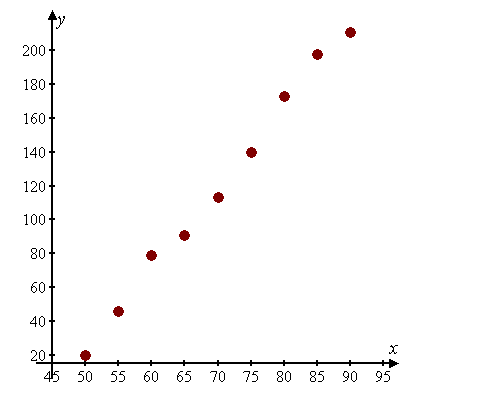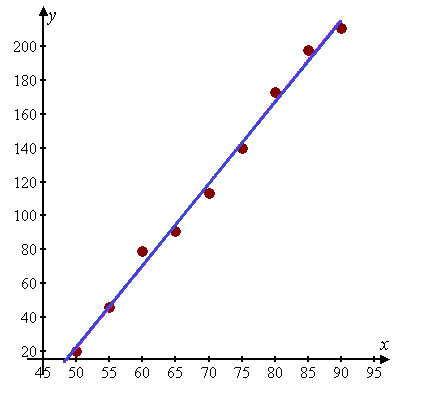# Make a scatter plot of the data.### Precalculus: Mathematics for Calcu...

6th Edition
Stewart + 5 others
Publisher: Cengage Learning
ISBN: 9780840068071### Precalculus: Mathematics for Calcu...

6th Edition
Stewart + 5 others
Publisher: Cengage Learning
ISBN: 9780840068071

#### Solutions

Chapter 1, Problem 5P

a.

To determine

## Make a scatter plot of the data.

Expert Solution### Explanation of Solution

Given information:

The chirping rate of crickets of a certain species appears to be related to temperature shown in table.

Temperature(F°)Chirpingrate(Chirpe/min)50205546607965917011375140801738519890211

Calculation:

Consider the given data table,

Temperature(F°)Chirpingrate(Chirpe/min)50205546607965917011375140801738519890211

The scatter plot is,Hence, the graph is plotted.

b.

To determine

### Find and graph the regression line.

Expert Solution

C=4.86T221### Explanation of Solution

Given information:

The chirping rate of crickets of a certain species appears to be related to temperature shown in table.

Temperature(F°)Chirpingrate(Chirpe/min)50205546607965917011375140801738519890211

Calculation:

It is observed from the scatter plot that all are linear,so linear model fits the data, by ising graphing calculator regression line will be,

C=4.86T221

Where C and T represents the chirping rate and temperature.

Now the plot for regression line is as follows,Hence, the graph is plotted and regression line is C=4.86T221 .

c.

To determine

### Use the linear model in part (b) to estimate the chirping rate at 100F .

Expert Solution

C=265chirps/min

### Explanation of Solution

Given information:

The chirping rate of crickets of a certain species appears to be related to temperature shown in table.

Temperature(F°)Chirpingrate(Chirpe/min)50205546607965917011375140801738519890211

Calculation:

The estimated chirping rate can be calculate bt putting T=100F in equation C=4.86T221 we get,

C=4.86(100)221=486221C=265

Hence, estimated chirping rate is, C=265chirps/min .

### Have a homework question?

Subscribe to bartleby learn! Ask subject matter experts 30 homework questions each month. Plus, you’ll have access to millions of step-by-step textbook answers!